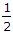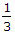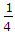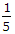# Civil Engineering - Surveying

### Exercise :: Surveying - Section 1

36.

The most reliable method of plotting a theodolite traverse, is

 A. by consecutive co-ordinates of each station B. by independent co-ordinates of each station C. by plotting included angles and scaling off each traverse leg D. by the tangent method of plotting.

Explanation:

No answer description available for this question. Let us discuss.

37.

The difference of level between a point below the plane of sight and one above, is the sum of two staff readings and an error would be produced equal to

 A. the distance between the zero of gradient and the foot of the staff B. twice the distance between the zero of graduation and the foot of the staff C. thrice the distance between the zero of graduation and the foot of the staff D. none of the above.

Explanation:

No answer description available for this question. Let us discuss.

38.

Offsets are measured with an accuracy of 1 in 40. If the point on the paper from both sources of error (due to angular and measurement errors) is not to exceed 0.05 cm on a scale of 1 cm = 20 m, the maximum length of offset should be limited to

 A. 14.14 B. 28.28 m C. 200 m D. none of these.

Explanation:

No answer description available for this question. Let us discuss.

39.

The probable error of the adjusted bearing at the middle is

 A.rn B.rn C.rn D.rn.

Explanation:

No answer description available for this question. Let us discuss.

40.

The bearings of the lines AB and BC are 146° 30' and 68° 30'. The included angle ABC is

 A. 102° B. 78° C. 45° D. none of these.